# Bryan Red 3/4

Locally sourced from limestone quarries in Hastings, Shakopee, and Bayport, this rock is a part of Minnesota and supports local industry. This low maintenance beautiful rock is a staple of Minnesota landscaping and history.

• Locally sourced
• Multiple sizes
• Our Smallest Bryan Red
• Beautiful red limestone
• Not to be used as contact lenses
If you need help finding out your total cubic yardage use one of our calculators below!
Aerial Map Calculator
Area Totals
Current Area
0 ft2
Total Area
0 ft2
Total Measurement: 0 cubic yards
Total Price: \$0
Square Footage Calculator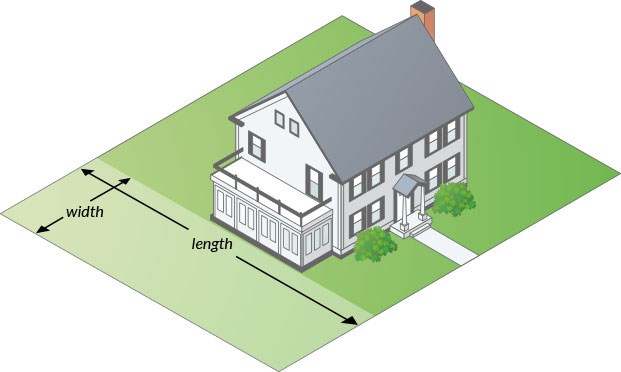#### How to measure your area.

• Area 1
• Area 1
• Area 1
Total Measurement: 0 cubic yards
Total Price: \$0

### How To Measure

#### Square or Rectangle

Measure the Length & Width and multiply.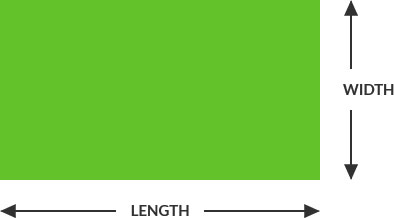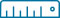Length x Width = Area
(12’ x 24’ = 288 ft2)

#### Right Triangle

Multiply the two sides that contact the right angle and divide by two.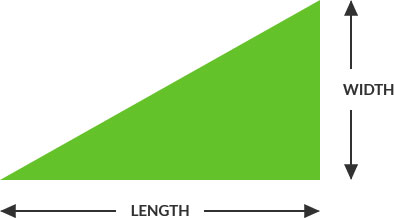Length x Width / 2 = Area
(12’ x 24’ = 288 ÷ 2 = 144 ft2)

#### Circular

Multiply the radius with the radius then multiply by 3.14. Radius is a straight line from the center of a circle to the outside of the circle.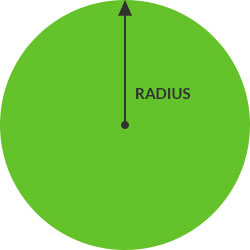##### Full Circle:(6’ x 6’ = 36 x 3.14 = 113 ft2)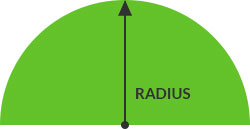##### Half Circle:Divide full circle radius by 2
(6’ x 6’ = 36 x 3.14 = 113 / 2 = 56.5 ft2)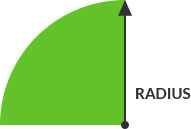##### Quarter Circle:Divide full circle radius by 4
(6’ x 6’ = 36 x 3.14 = 113 / 4 = 28.25 ft2)